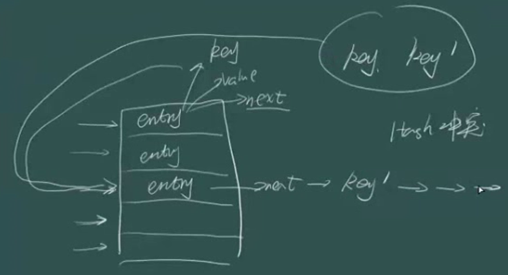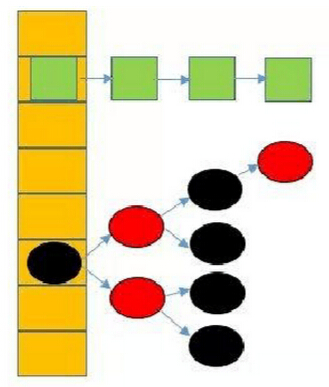JDK7与JDK8中HashMap的实现

JDK7中的HashMap

HashMap底层维护一个数组，数组中的每一项都是一个Entry

transient Entry<K,V>[] table;

static class Entry<K,V> implements Map.Entry<K,V> {
final K key;
V value;
Entry<K,V> next;
int hash;

final int hash(Object k) {
int h = 0;
h ^= k.hashCode();

h ^= (h >>> 20) ^ (h >>> 12);
return h ^ (h >>> 7) ^ (h >>> 4);
}

static int indexFor(int h, int length) {
return h & (length-1);
}private V putForNullKey(V value) {
for (Entry<K,V> e = table; e != null; e = e.next) {
if (e.key == null) {
V oldValue = e.value;
e.value = value;
e.recordAccess(this);
return oldValue;
}
}
modCount++;
addEntry(0, null, value, 0);
return null;
}

void addEntry(int hash, K key, V value, int bucketIndex) {
if ((size >= threshold) && (null != table[bucketIndex])) {
resize(2 * table.length);
hash = (null != key) ? hash(key) : 0;
bucketIndex = indexFor(hash, table.length);
}

createEntry(hash, key, value, bucketIndex);
}

jdk7中resize，只有当 size>=threshold并且 table中的那个槽中已经有Entry时，才会发生resize。即有可能虽然size>=threshold，但是必须等到每个槽都至少有一个Entry时，才会扩容。还有注意每次resize都会扩大一倍容量

JDK8中的HashMap

JDK7中HashMap采用的是位桶+链表的方式，即我们常说的散列链表的方式，而JDK8中采用的是位桶+链表/红黑树（有关红黑树请查看红黑树）的方式，也是非线程安全的。当某个位桶的链表的长度达到某个阀值的时候，这个链表就将转换成红黑树。JDK8中，当同一个hash值的节点数不小于8时，将不再以单链表的形式存储了，会被调整成一颗红黑树（上图中null节点没画）。这就是JDK7与JDK8中HashMap实现的最大区别。

JDK中Entry的名字变成了Node，原因是和红黑树的实现TreeNode相关联。

transient Node<K,V>[] table;

static final int TREEIFY_THRESHOLD = 8;

public V put(K key, V value) {
return putVal(hash(key), key, value, false, true);
}

final V putVal(int hash, K key, V value, boolean onlyIfAbsent,
boolean evict) {
Node<K,V>[] tab;
Node<K,V> p;
int n, i;
//如果当前map中无数据，执行resize方法。并且返回n
if ((tab = table) == null || (n = tab.length) == 0)
n = (tab = resize()).length;
//如果要插入的键值对要存放的这个位置刚好没有元素，那么把他封装成Node对象，放在这个位置上就完事了
if ((p = tab[i = (n - 1) & hash]) == null)
tab[i] = newNode(hash, key, value, null);
//否则的话，说明这上面有元素
else {
Node<K,V> e; K k;
//如果这个元素的key与要插入的一样，那么就替换一下，也完事。
if (p.hash == hash &&
((k = p.key) == key || (key != null && key.equals(k))))
e = p;
//1.如果当前节点是TreeNode类型的数据，执行putTreeVal方法
else if (p instanceof TreeNode)
e = ((TreeNode<K,V>)p).putTreeVal(this, tab, hash, key, value);
else {
//还是遍历这条链子上的数据，跟jdk7没什么区别
for (int binCount = 0; ; ++binCount) {
if ((e = p.next) == null) {
p.next = newNode(hash, key, value, null);
//2.完成了操作后多做了一件事情，判断，并且可能执行treeifyBin方法
if (binCount >= TREEIFY_THRESHOLD - 1) // -1 for 1st
treeifyBin(tab, hash);
break;
}
if (e.hash == hash &&
((k = e.key) == key || (key != null && key.equals(k))))
break;
p = e;
}
}
if (e != null) { // existing mapping for key
V oldValue = e.value;
if (!onlyIfAbsent || oldValue == null) //true || --
e.value = value;
//3.
afterNodeAccess(e);
return oldValue;
}
}
++modCount;
//判断阈值，决定是否扩容
if (++size > threshold)
resize();
//4.
afterNodeInsertion(evict);
return null;
}

treeifyBin()就是将链表转换成红黑树。

static final int hash(Object key) {
int h;
return (key == null) ? 0 : (h = key.hashCode()) ^ (h >>> 16);
}

Reference：

1. http://www.tuicool.com/articles/Yruqiye